Problems on finding the G.P. when certain terms or conditions are given are included in this exercise. For any clarifications on how to solve problems of this chapter and others, students can refer to the Selina Solutions for Class 10 Maths anytime. It is a vital resource for exam preparations. Further, the solutions to this exercise can be accessed in the Selina Solutions Concise Maths Class 10 Chapter 11 Geometric Progression Exercise 11(B) PDF, which is given in the links below.

## Selina Solutions Concise Maths Class 10 Chapter 11 Geometric Progression Exercise 11(B) Download PDF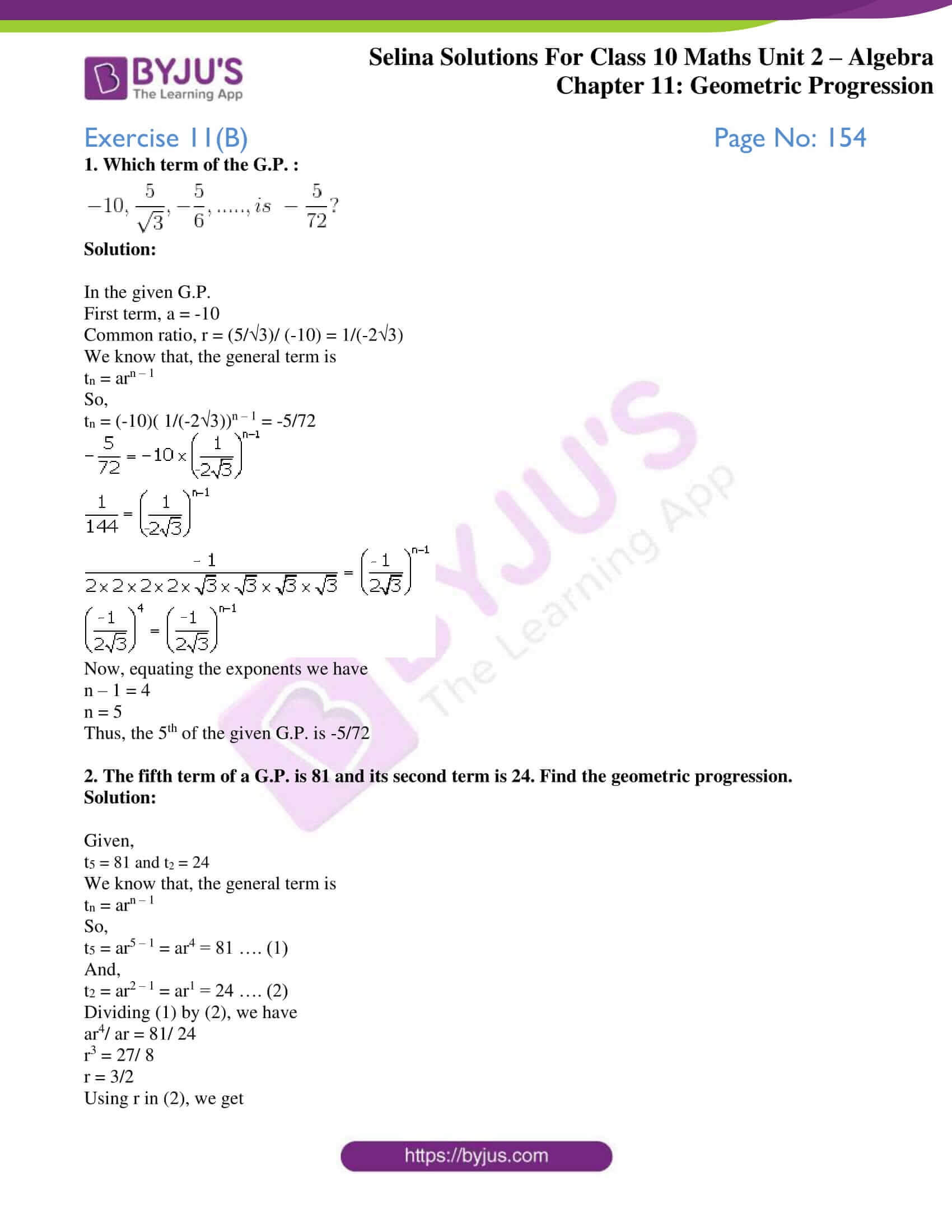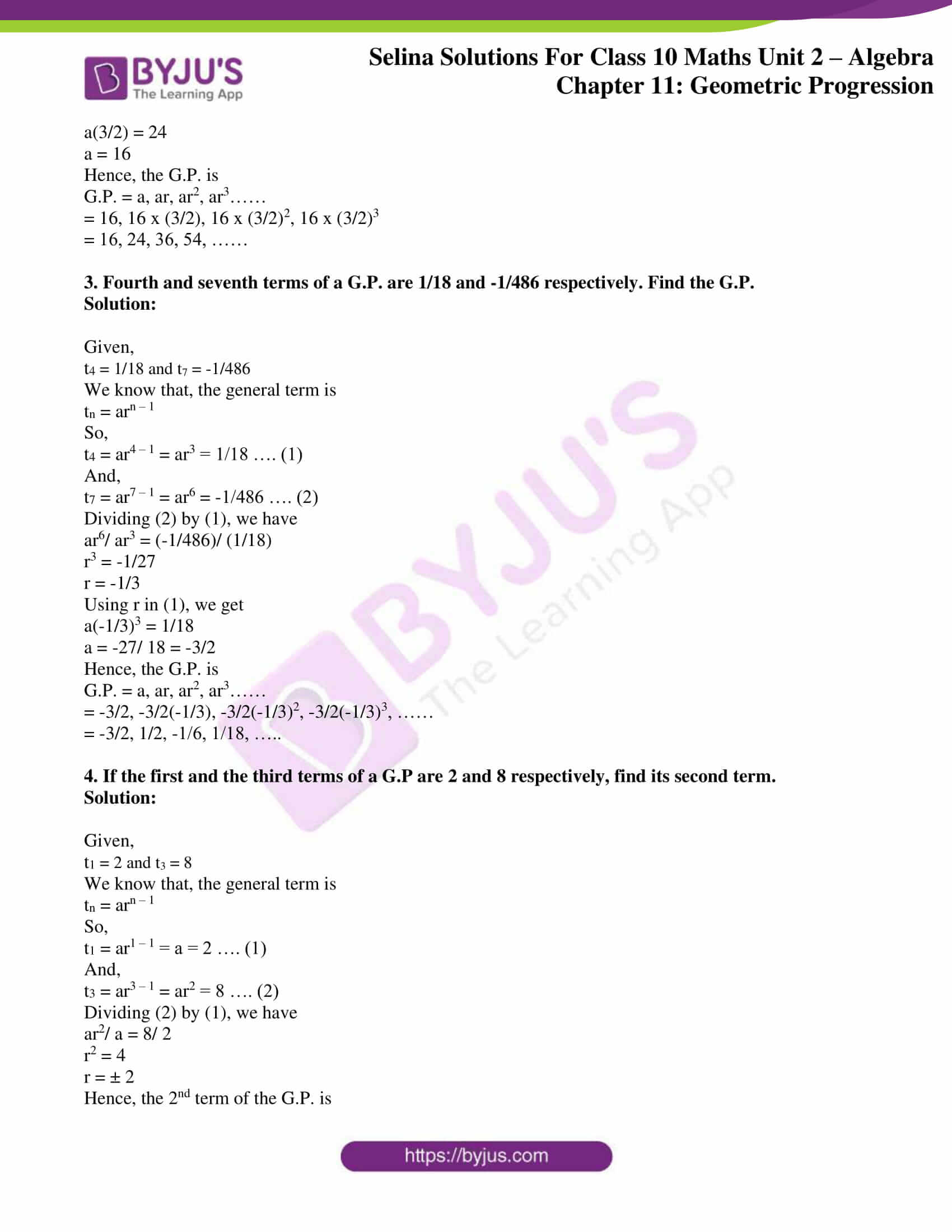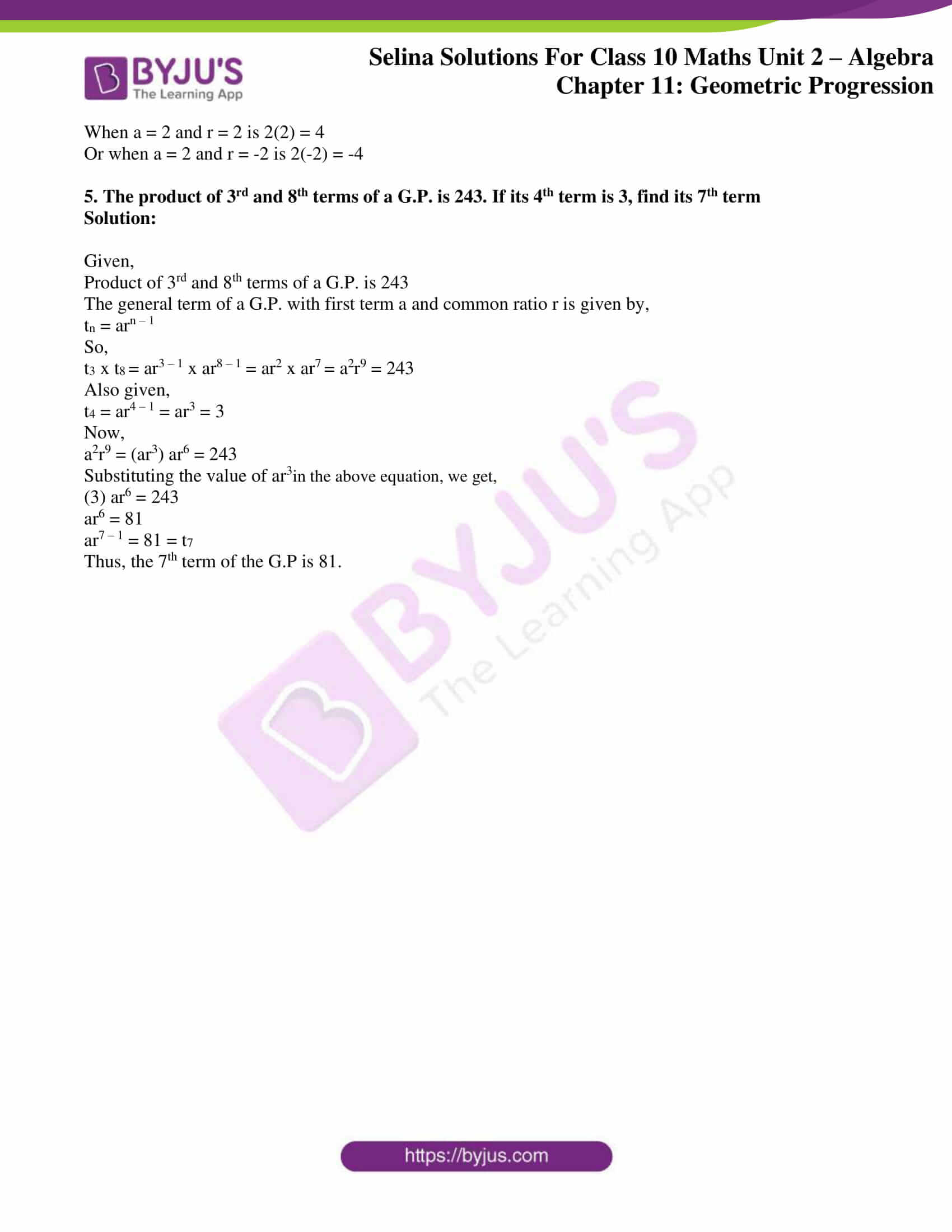### Access Selina Solutions Concise Maths Class 10 Chapter 11 Geometric Progression Exercise 11(B)

#### Exercise 11(B) Page No: 154

1. Which term of the G.P. :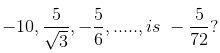Solution:

In the given G.P.

First term, a = -10

Common ratio, r = (5/√3)/ (-10) = 1/(-2√3)

We know that, the general term is

tn = arn – 1

So,

tn = (-10)( 1/(-2√3))n – 1 = -5/72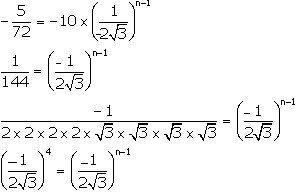Now, equating the exponents we have

n – 1 = 4

n = 5

Thus, the 5th of the given G.P. is -5/72

2. The fifth term of a G.P. is 81 and its second term is 24. Find the geometric progression.

Solution:

Given,

t5 = 81 and t2 = 24

We know that, the general term is

tn = arn – 1

So,

t5 = ar5 – 1 = ar4 = 81 …. (1)

And,

t2 = ar2 – 1 = ar1 = 24 …. (2)

Dividing (1) by (2), we have

ar4/ ar = 81/ 24

r3 = 27/ 8

r = 3/2

Using r in (2), we get

a(3/2) = 24

a = 16

Hence, the G.P. is

G.P. = a, ar, ar2, ar3……

= 16, 16 x (3/2), 16 x (3/2)2, 16 x (3/2)3

= 16, 24, 36, 54, ……

3. Fourth and seventh terms of a G.P. are 1/18 and -1/486 respectively. Find the G.P.

Solution:

Given,

t4 = 1/18 and t7 = -1/486

We know that, the general term is

tn = arn – 1

So,

t4 = ar4 – 1 = ar3 = 1/18 …. (1)

And,

t7 = ar7 – 1 = ar6 = -1/486 …. (2)

Dividing (2) by (1), we have

ar6/ ar3 = (-1/486)/ (1/18)

r3 = -1/27

r = -1/3

Using r in (1), we get

a(-1/3)3 = 1/18

a = -27/ 18 = -3/2

Hence, the G.P. is

G.P. = a, ar, ar2, ar3……

= -3/2, -3/2(-1/3), -3/2(-1/3)2, -3/2(-1/3)3, ……

= -3/2, 1/2, -1/6, 1/18, …..

4. If the first and the third terms of a G.P are 2 and 8 respectively, find its second term.

Solution:

Given,

t1 = 2 and t3 = 8

We know that, the general term is

tn = arn – 1

So,

t1 = ar1 – 1 = a = 2 …. (1)

And,

t3 = ar3 – 1 = ar2 = 8 …. (2)

Dividing (2) by (1), we have

ar2/ a = 8/ 2

r2 = 4

r = ± 2

Hence, the 2nd term of the G.P. is

When a = 2 and r = 2 is 2(2) = 4

Or when a = 2 and r = -2 is 2(-2) = -4

5. The product of 3rd and 8th terms of a G.P. is 243. If its 4th term is 3, find its 7th term

Solution:

Given,

Product of 3rd and 8th terms of a G.P. is 243

The general term of a G.P. with first term a and common ratio r is given by,

tn = arn – 1

So,

t3 x t8 = ar3 – 1 x ar8 – 1 = ar2 x ar7 = a2r9 = 243

Also given,

t4 = ar4 – 1 = ar3 = 3

Now,

a2r9 = (ar3) ar6 = 243

Substituting the value of ar3in the above equation, we get,

(3) ar6 = 243

ar6 = 81

ar7 – 1 = 81 = t7

Thus, the 7th term of the G.P is 81.

### Access other exercises of Selina Solutions Concise Maths Class 10 Chapter 11 Geometric Progression

Exercise 11(A) Solutions

Exercise 11(C) Solutions

Exercise 11(D) Solutions